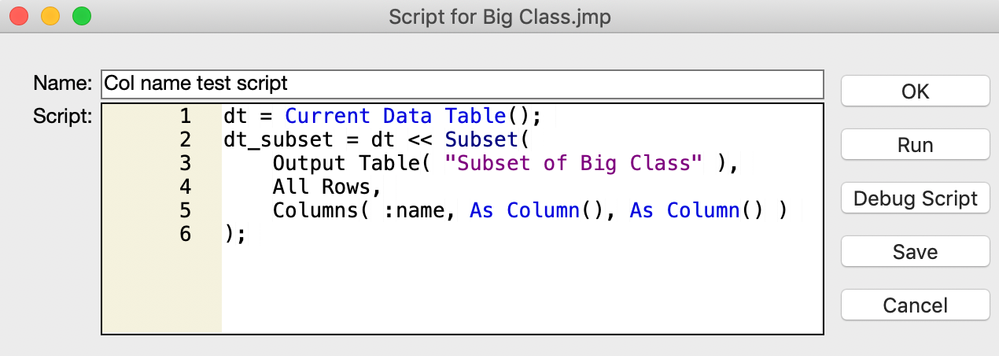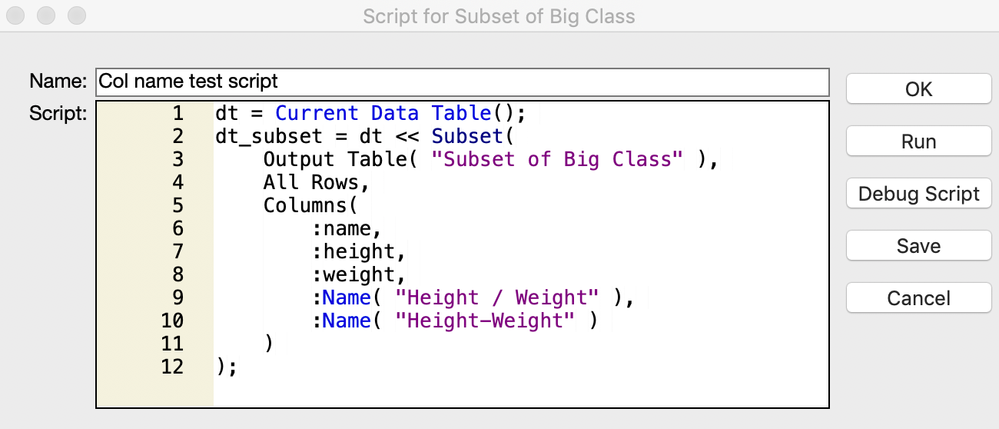Choose Language Hide Translation Bar
Highlighted

## What is the recommended format to specify column names for a subset in JSL?

When creating a subset table in JSL, column names can most simply be specified with a colon character before the name, as in `:height`.  However, if that name has a dash ('-')  character in it, that doesn't work.  But I find that adding quotes around the name gets it to work.  The complete script below demonstrates both methods (though `Big Class.jmp` doesn't contain any column names with dashes).

``````Names Default To Here( 1 );
dt = Open( "\$SAMPLE_DATA/Big Class.jmp" );

scrName = "Col name test script";

dt << New Script(  // (use of 'New Script' gets script written to data table.)
scrName,
//---- create subset with StartTime and given FOMs ----
dt = Current Data Table();
dt_subset = dt << Subset(
Output Table( "Subset of Big Class" ),
All Rows,
Columns(
:name,		// not in quotes.
:"height",	// in quotes -- required if col name contains '-'.
:"weight"	// in quotes -- required if col name contains '-'.
);
);
);

// run script to generate graph.
dt << Run Script( scrName );``````

And, as desired, this script gets added to the JMP table with the name `Col name test script`.  And executing that script from within the JMP table (by selecting its green arrow) properly regenerates the subset table.  So far, so good.

But funny things happen if you want to go in and start editing that script (by double-clicking on the script name and opening up the editor).  In this case, running the script, as is, from within the script editor fails to add the quoted column names to the subset table.Looking at the script, it seems that double quotes cause column names to get treated as null `As Column` specifications.  (It's very strange that the script runs ok from the green arrow, but not from within the script editor;  perhaps it's saved internally one way, but gets rendered out to the editor differently??)

In any case, my question is:  Is there a recommended way to specify subset column names generally to protect against special characters (like dashes) in the names, but still be able to use the script editor to develop and debug a script?  (I'm actually generating the `New Script` code programmatically, so I don't know ahead of time whether any special characters exist in the names.)  I'm running JMP 14.3.0 on Mac OS.

1 ACCEPTED SOLUTION

Accepted Solutions
Highlighted

## Re: What is the recommended format to specify column names for a subset in JSL?

The proper way to reference column names that have special characters in them is with the :Name() function

``````names default to here(1);
dt=open("\$SAMPLE_DATA/big class.jmp");

dt << new column("Height / Weight", formula(:height / :weight));

colList = dt << get column names();

show( colList );
/*
colList = {name, age, sex, height, weight, Name("Height / Weight")};
*/

dt << Distribution( Continuous Distribution( Column( colList ) ) );

show(:Name("height / weight"));``````
Jim
2 REPLIES 2
Highlighted

## Re: What is the recommended format to specify column names for a subset in JSL?

The proper way to reference column names that have special characters in them is with the :Name() function

``````names default to here(1);
dt=open("\$SAMPLE_DATA/big class.jmp");

dt << new column("Height / Weight", formula(:height / :weight));

colList = dt << get column names();

show( colList );
/*
colList = {name, age, sex, height, weight, Name("Height / Weight")};
*/

dt << Distribution( Continuous Distribution( Column( colList ) ) );

show(:Name("height / weight"));``````
Jim
Highlighted

## Re: What is the recommended format to specify column names for a subset in JSL?

Yes, that seems to make things work well.  Thank you so much, Jim!

For completeness, here is how it turned out.

First, the script that saves a script to the `Big Class.jmp`data table:

``````Names Default To Here( 1 );
dt = Open( "\$SAMPLE_DATA/Big Class.jmp" );

dt << New Column("Height / Weight", Formula(:height / :weight));
dt << New Column("Height-Weight",   Formula(:height / :weight));  // using '-'.

scrName = "Col name test script";

dt << New Script(  // (use of 'New Script' gets script written to data table.)
scrName,
// create subset.
dt = Current Data Table();
dt_subset = dt << Subset(
Output Table( "Subset of Big Class" ),
All Rows,
Columns(
:name,
:Name("height"),
:Name("weight"),
:Name("Height / Weight"),
:Name("Height-Weight")
);
);
);

// run script to create subset.
dt << Run Script( scrName );``````

And then, opening up the newly created script in `Big Class.jmp`:And, unlike before, when I run this script from this window, it runs as expected.

Article Labels

There are no labels assigned to this post.